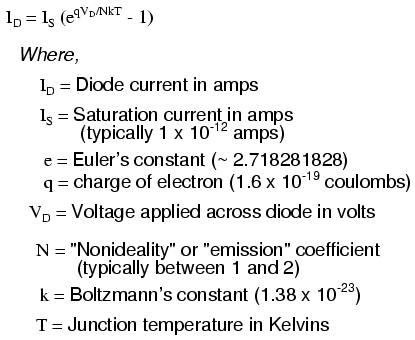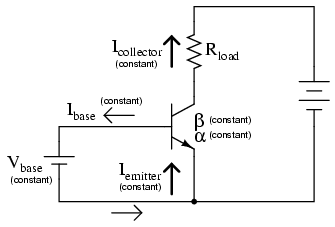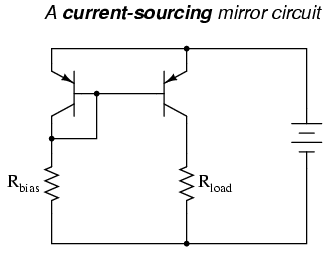# current mirror

## Current mirrors

An interesting and often-used circuit applying the bipolar junction transistor is the so-called current mirror, which serves as a simple current regulator, supplying nearly constant current to a load over a wide range of load resistances.

We know that in a transistor operating in its active mode, collector current is equal to base current multiplied by the ratio β. We also know that the ratio between collector current and emitter current is called α. Because collector current is equal to base current multiplied by β, and emitter current is the sum of the base and collector currents, α should be mathematically derivable from β. If you do the algebra, you’ll find that α = β/(β+1) for any transistor.

We’ve seen already how maintaining a constant base current through an active transistor results in the regulation of collector current, according to the β ratio. Well, the α ratio works similarly: if emitter current is held constant, collector current will remain at a stable, regulated value so long as the transistor has enough collector-to-emitter voltage drop to maintain it in its active mode. Therefore, if we have a way of holding emitter current constant through a transistor, the transistor will work to regulate collector current at a constant value.

Remember that the base-emitter junction of a BJT is nothing more than a PN junction, just like a diode, and that the “diode equation” specifies how much current will go through a PN junction given forward voltage drop and junction temperature:If both junction voltage and temperature are held constant, then the PN junction current will likewise be constant. Following this rationale, if we were to hold the base-emitter voltage of a transistor constant, then its emitter current should likewise be constant, given a constant temperature:This constant emitter current, multiplied by a constant α ratio, gives a constant collector current through Rload, provided that there is enough battery voltage to keep the transistor in its active mode for any change in Rload‘s resistance.

Maintaining a constant voltage across the transistor’s base-emitter junction is easy: use a forward-biased diode to establish a constant voltage of approximately 0.7 volts, and connect it in parallel with the base-emitter junction:

Now, here’s where it gets interesting. The voltage dropped across the diode probably won’t be 0.7 volts exactly. The exact amount of forward voltage dropped across it depends on the current through the diode, and the diode’s temperature, all in accordance with the diode equation. If diode current is increased (say, by reducing the resistance of Rbias), its voltage drop will increase slightly, increasing the voltage drop across the transistor’s base-emitter junction, which will increase the emitter current by the same proportion, assuming the diode’s PN junction and the transistor’s base-emitter junction are well-matched to each other. In other words, transistor emitter current will closely equal diode current at any given time. If you change the diode current by changing the resistance value of Rbias, then the transistor’s emitter current will follow suit, because the emitter current is described by the same equation as the diode’s, and both PN junctions experience the same voltage drop.

Remember, the transistor’s collector current is almost equal to its emitter current, as the α ratio of a typical transistor is almost unity (1). If we have control over the transistor’s emitter current by setting diode current with a simple resistor adjustment, then we likewise have control over the transistor’s collector current. In other words, collector current mimics, or mirrors, diode current.

Current through resistor Rload is therefore a function of current set by the bias resistor, the two being nearly equal. This is the function of the current mirror circuit: to regulate current through the load resistor by conveniently adjusting the value of Rbias. It is very easy to create a set amount of diode current, as current through the diode is described by a simple equation: power supply voltage minus diode voltage (almost a constant value), divided by the resistance of Rbias.

To better match the characteristics of the two PN junctions (the diode junction and the transistor base-emitter junction), a transistor may be used in place of a regular diode, like this:Because temperature is a factor in the “diode equation,” and we want the two PN junctions to behave identically under all operating conditions, we should maintain the two transistors at exactly the same temperature. This is easily done using discrete components by gluing the two transistor cases back-to-back. If the transistors are manufactured together on a single chip of silicon (as a so-called integrated circuit, or IC), the designers should locate the two transistors very close to one another to facilitate heat transfer between them.

The current mirror circuit shown with two NPN transistors is sometimes called a current-sinking type, because the regulating transistor conducts current to the load from ground (“sinking” current), rather than from the positive side of the battery (“sourcing” current). If we wish to have a grounded load, and a current sourcing mirror circuit, we could use PNP transistors like this:REVIEW:
A current mirror is a transistor circuit that regulates current through a load resistance, the regulation point being set by a simple resistor adjustment.
Transistors in a current mirror circuit must be maintained at the same temperature for precise operation. When using discrete transistors, you may glue their cases together to help accomplish this.
Current mirror circuits may be found in two basic varieties: the current sinking configuration, where the regulating transistor connects the load to ground; and the current sourcing configuration, where the regulating transistor connects the load to the positive terminal of the DC power supply.

## Transistor ratings and packages

*** INCOMPLETE ***

Like all eletrical and electronic components, transistors are limited in the amounts of voltage and current they can handle without sustaining damage. Since transistors are a bit more complex than some of the other components you’re used to seeing at this point, they tend to have more kinds of ratings. What follows is an itemized description of some typical transistor ratings.

Power dissipation: When a transistor conducts current between collector and emitter, it also drops voltage between those two points. At any given time, the power dissipated by a transistor is equal to the product (multiplication) of collector current and collector-emitter voltage. Just like resistors, transistors are rated in terms of how many watts they can safely dissipate without sustaining damage. High temperature is the mortal enemy of all semiconductor devices, and bipolar transistors tend to be more susceptible to thermal damage than most. Power ratings are always given in reference to the temperature of ambient (surrounding) air. When transistors are to be used in hotter-than-normal environments, their power ratings must be derated to avoid a shortened service life.

Reverse voltages: As with diodes, bipolar transistors are rated for maximum allowable reverse-bias voltage across their PN junctions. This includes voltage ratings for the base-emitter junction, base-collector junction, and also from collector to emitter. The rating for maximum collector-emitter voltage can be thought of in terms of the maximum voltage it can withstand while in full-cutoff mode (no base current). This rating is of particular importance when using a bipolar transistor as a switch.

Collector current: A maximum value for collector current will be given by the manufacturer in amps. Understand that this maximum figure assumes a saturated state (minimum collector-emitter voltage drop). If the transistor is not saturated, and in fact is dropping substantial voltage between collector and emitter, the maximum power dissipation rating will probably be exceeded before the maximum collector current rating will. Just something to keep in mind when designing a transistor circuit!

Saturation voltages: Ideally, a saturated transistor acts as a closed switch contact between collector and emitter, dropping zero voltage at full collector current. In reality this is never true. Manufacturers will specify the maximum voltage drop of a transistor at saturation, both between the collector and emitter, and also between base and emitter (forward voltage drop of that PN junction). Collector-emitter voltage drop at saturation is generally expected to be 0.3 volts or less, but this figure is of course dependent on the specific type of transistor. Base-emitter forward voltage drop is very similar to that of an equivalent diode, which should come as no surprise.

Beta: The ratio of collector current to base current, β is the fundamental parameter characterizing the amplifying ability of a bipolar transistor. β is usually assumed to be a constant figure in circuit calculations, but unfortunately this is far from true in practice. As such, manufacturers provide a set of β (or “hfe“) figures for a given transistor over a wide range of operating conditions, usually in the form of maximum/minimum/typical ratings. It may surprise you to see just how widely β can be expected to vary within normal operating limits. One popular small-signal transistor, the 2N3903, is advertised as having a β ranging from 15 to 150 depending on the amount of collector current. Generally, β is highest for medium collector currents, decreasing for very low and very high collector currents.

Alpha: the ratio of collector current to emitter current, α may be derived from β, being equal to β/(β+1).

Bipolar transistors come in a wide variety of physical packages. Package type is primarily dependent upon the power dissipation of the transistor, uch like resistors: the greater the maximum power dissipation, the larger the device has to be to stay cool. There are several standardized package types for three-terminal semiconductor devices, any of which may be used to house a bipolar transistor. This is an important fact to consider: there are many other semiconductor devices other than bipolar transistors which have three connection points. It is impossible to positively identify a three-terminal semiconductor device without referencing the part number printed on it, and/or subjecting it to a set of electrical tests.

Related

COMMENT songs